0
4
1
1

# TiFlash 面向编译器的自动向量化加速YY-ha  发表于  2022-06-28

• SIMD 介绍
• SIMD 函数派发方案
• 面向编译器的优化

# SIMD 介绍

SIMD 是重要的重要的程序加速手段。CMU DB 组在 Advanced Database Systems 中有专门的两个章节（vectorization-1, vectorization-2）介绍 SIMD 向量化在数据库中的应用，可见其对现代数据库系统的重要性。本文章简要介绍一些在 TiFlash 中使用编译器进行自动向量化所需要的入门知识。

TiFlash 目前支持的架构是 x86-64 和 Aarch64，操作系统平台有 Linux 和 MacOS。受制于平台 ISA 和操作系统 API，在不同环境中做 SIMD 支持会遇到不同的麻烦。

## X86-64

• x86-64: CMOV, CMPXCHG8B, FPU, FXSR, MMX, FXSR, SCE, SSE, SSE2
• x86-64-v2: (close to Nehalem) CMPXCHG16B, LAHF-SAHF, POPCNT, SSE3, SSE4.1, SSE4.2, SSSE3
• x86-64-v3: (close to Haswell) AVX, AVX2, BMI1, BMI2, F16C, FMA, LZCNT, MOVBE, XSAVE
• x86-64-v4: AVX512F, AVX512BW, AVX512CD, AVX512DQ, AVX512VL

x86-64 上不同 CPU 架构之前相同拓展指令集的开销也是不同的，一般来说，可以在 Intel Intrinsic Guide 上简要查看相关指令在不同微架构上的 CPI 信息。而如果要针对具体的平台优化，则可以阅读平台相关的 Tuning Guides and Performance Analysis PapersINTEL® ADVANCED VECTOR EXTENSIONS 以及 Intel® 64 and IA-32 Architectures Software Developer Manuals （Software Optimization Reference Manual 系列）来获得 Intel 官方的建议。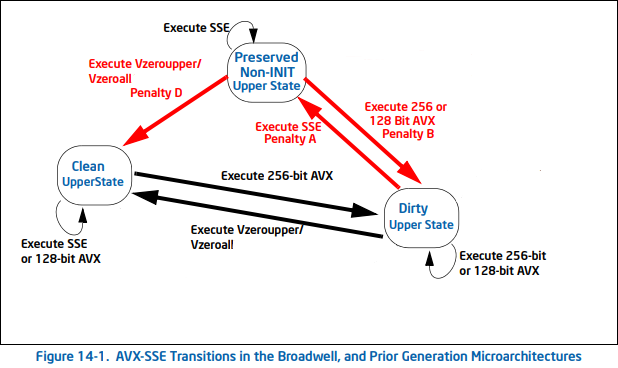``````#!/usr/bin/env bash

args=(-batch -ex "file \$1")
do
args+=("-ex" "disassemble '\$line'")
done < <(nm --demangle \$1 | grep \$2 | cut -d\  -f3-)
gdb "\${args[@]}" | c++filt

# bash ./this-script.sh tiflash xxx
``````
``````#!/usr/bin/env bash

# LLDB version
args=(--batch -o "file \$1")
do
args+=("-o" "disassemble -F intel -n '\$line'")
done < <(nm --defined-only --demangle \$1 | grep \$2 | cut -d\  -f3-)

lldb "\${args[@]}" | c++filt
# bash ./this-script.sh tiflash xxx
``````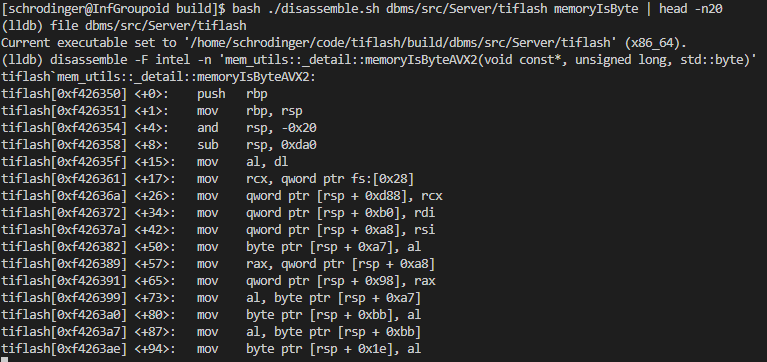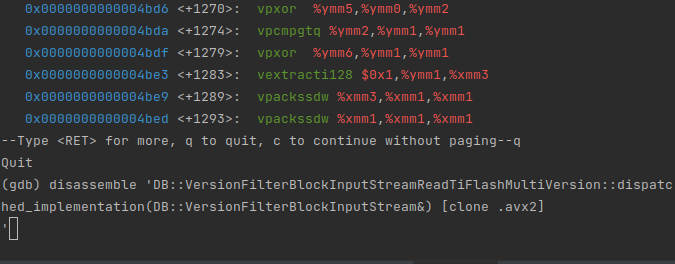## Aarch64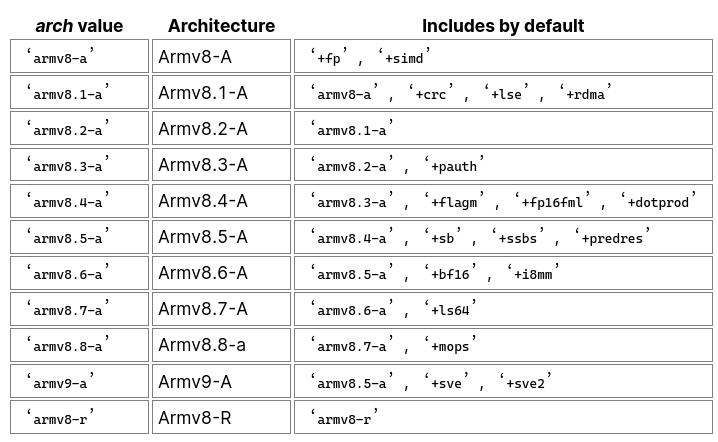ASIMD 描述的是定长向量化操作，作用于 64bit 和 128bit 的寄存器，功能上和 SSE 系列接近。SVE 则是使用变长向量，Vendor 可以提供最高到 2048bit 的超宽寄存器。使用 Per-Lane Prediction 的方案，SVE 指令集建立了一种无需知道实际寄存器宽度的编程模型。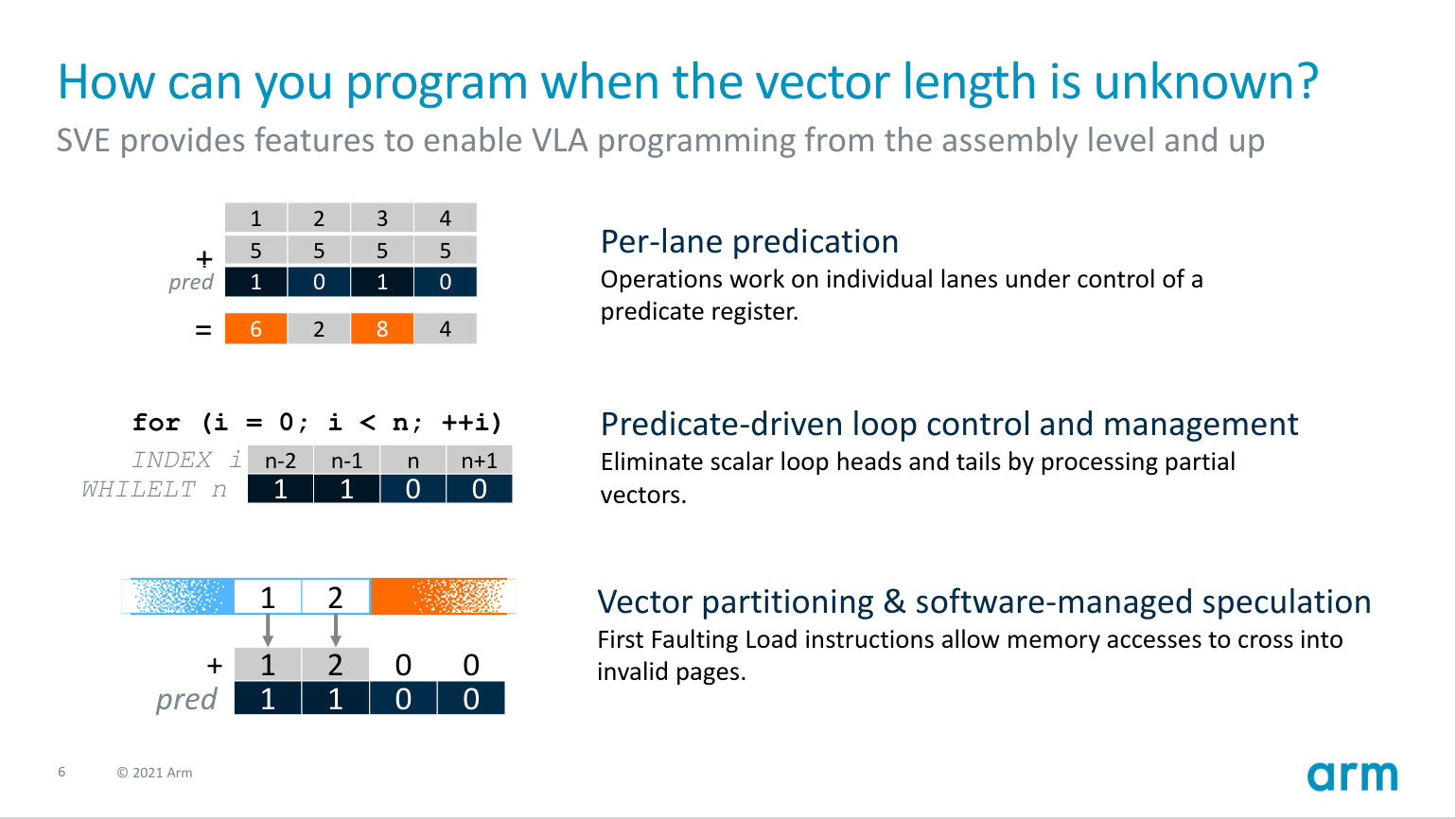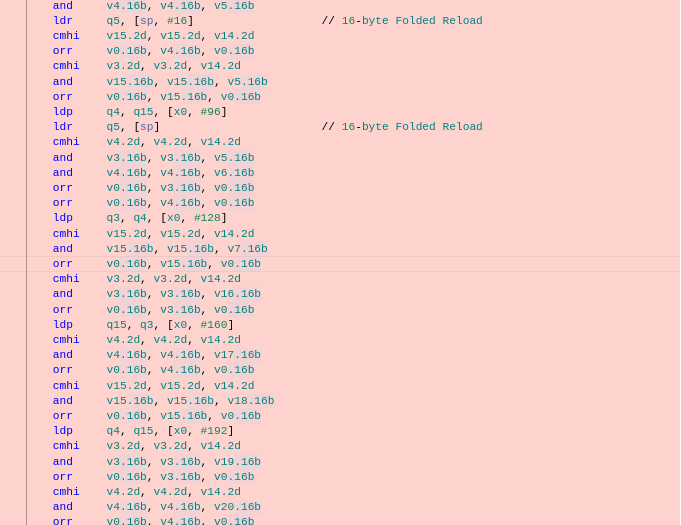# SIMD 函数派发方案

TiFlash 的 CD Pipeline 对于每种OS/Arch组合生成一个统一的二进制文件包进行发布，因此整体编译的目标都是相对通用的架构。而 SIMD 指令集在不同平台具有差异性，因此我们需要一些方案来派发被向量化的函数。以下提供两大类方案，运行时和加载时。整体来说，可以参考以下条件来选择：

• 如果想支持非 Linux 目标，且已知操作本身用时相对较多，不在乎多一两个 branch，可以使用运行时的派发。在这种情况下，TiFlash 里有提供对应向量化方案的运行时开关，功能更可控 。
• 如果操作极其大量地被使用，且 branch 可能会影响性能，可以优先考虑加载时派发。TiFlash 在生产环境中基本上使用 Linux，所以可以只为 MacOS 提供默认版本的函数。

## 运行时派发

``````__attribute__((target("avx512f"))) void test4096_avx512(bool * __restrict a, const int * __restrict b)
{
for (int i = 0; i < 4096; ++i)
{
a[i] = b[i] > 0;
}
}

__attribute__((target("avx2"))) void test4096_avx2(bool * __restrict a, const int * __restrict b)
{
for (int i = 0; i < 4096; ++i)
{
a[i] = b[i] > 0;
}
}

__attribute__((noinline)) void test4096_generic(bool * __restrict a, const int * __restrict b)
{
for (int i = 0; i < 4096; ++i)
{
a[i] = b[i] > 0;
}
}

void test4096(bool * __restrict a, const int * __restrict b)
{
if (common::cpu_feature_flags.avx512f)
{
return test4096_avx512(a, b);
}
if (common::cpu_feature_flags.avx2)
{
return test4096_avx2(a, b);
}
return test4096_generic(a, b);
}
``````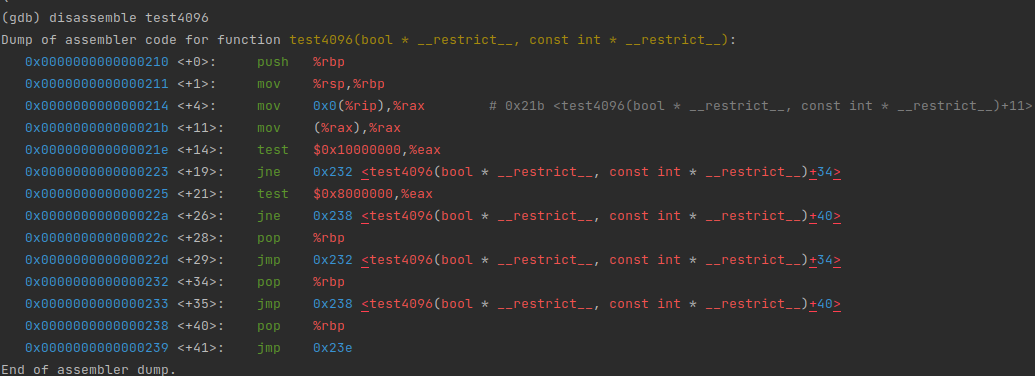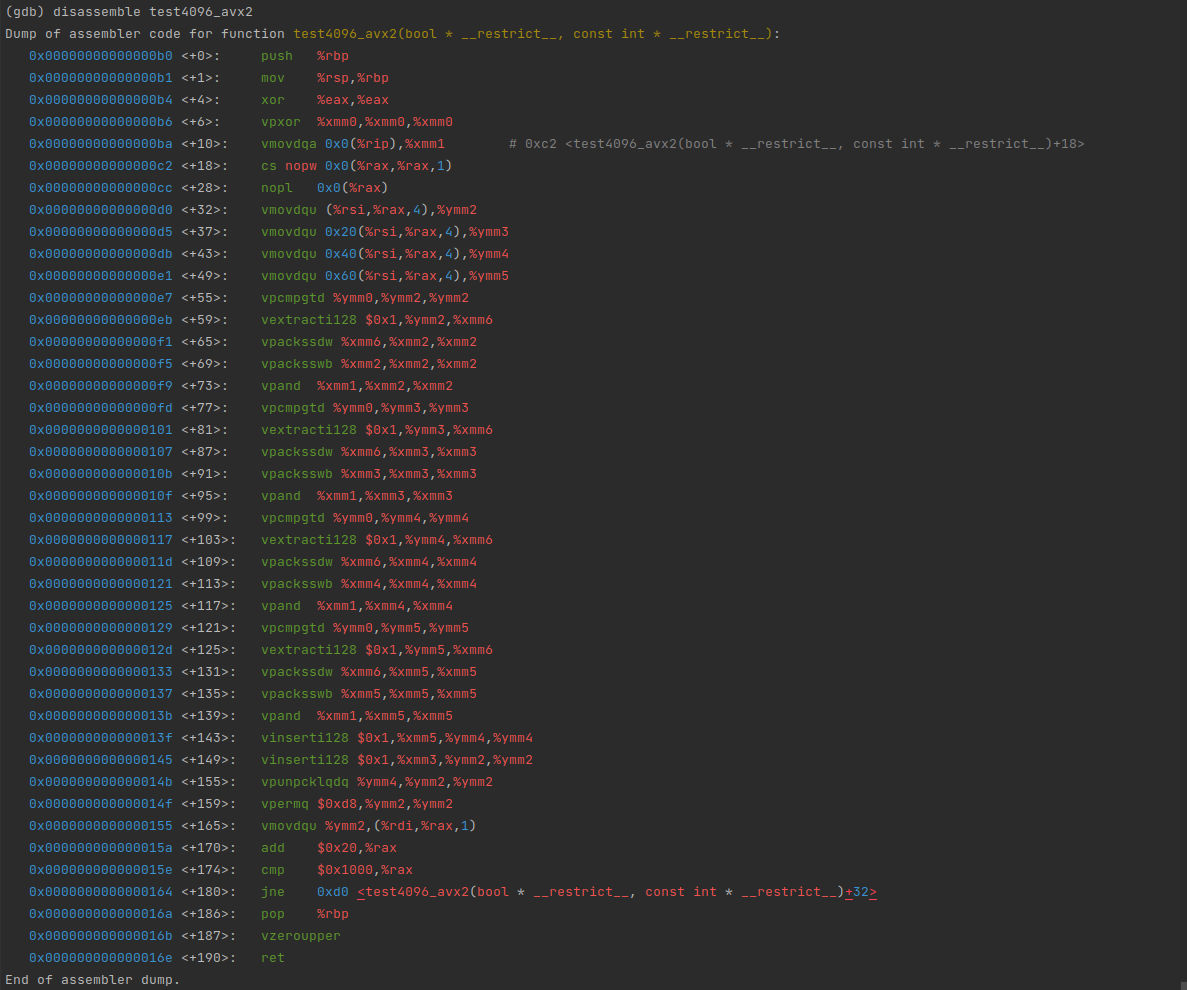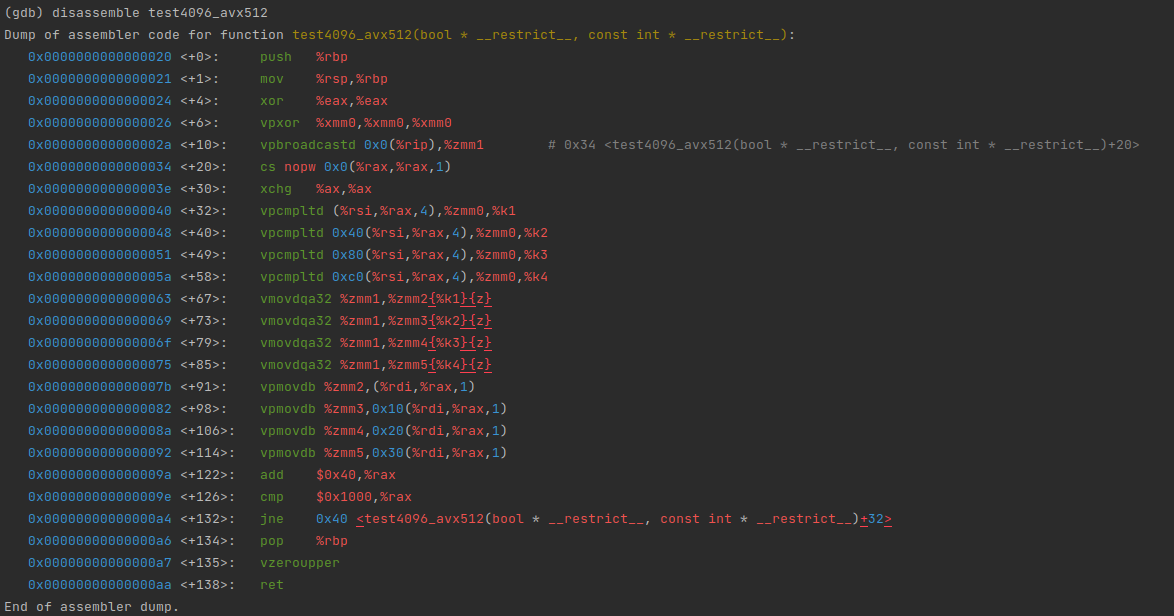``````#include <Common/TargetSpecific.h>
TIFLASH_DECLARE_MULTITARGET_FUNCTION(
/* return type */ void,
/* function name */ test4096,
/* argument names */ (a, b),
/* argument list */ (bool * __restrict a, const int * __restrict b),
/* body */ {
for (int i = 0; i < 4096; ++i)
{
a[i] = b[i] > 0;
}
})
``````

## IFUNC 派发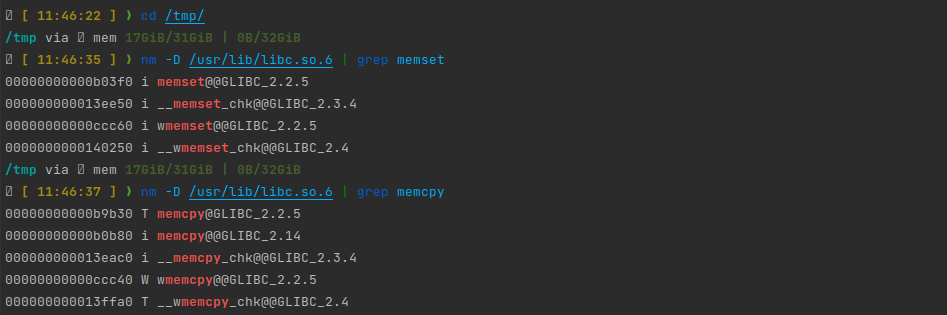`test4096`可以改写：

``````void test4096(bool * __restrict a, const int * __restrict b) __attribute__((ifunc("test4096_resolver")));
extern "C" void * test4096_resolver()
{
if (__builtin_cpu_supports("avx512f"))
return reinterpret_cast<void *>(&test4096_avx512);
if (__builtin_cpu_supports("avx2"))
return reinterpret_cast<void *>(&test4096_avx2);
return reinterpret_cast<void *>(&test4096_generic);
}
``````

1. 仅适用于 GNU/Linux 平台
1. ifunc 的 resolver 必须在当前 unit 内。如果 resolver 是 c++ 的函数，需要提供 mangle 后的名字。
1. resolver 执行于进入 C 运行时和 C++ 运行时之前，不能用 TiFlash 的检测功能。在`x86_64` 平台，可以使用 `__builtin_cpu_supports`; 在 `aarch64` 上，可以使用以下方案：

``````#include <sys/auxv.h>
#ifndef HWCAP2_SVE2
#define HWCAP2_SVE2 (1 << 1)
#endif

#ifndef HWCAP_SVE
#define HWCAP_SVE (1 << 22)
#endif

#ifndef AT_HWCAP2
#define AT_HWCAP2 26
#endif

#ifndef AT_HWCAP
#define AT_HWCAP 16
#endif

namespace detail
{
static inline bool sve2_supported()
{
auto hwcaps = getauxval(AT_HWCAP2);
return (hwcaps & HWCAP2_SVE2) != 0;
}

static inline bool sve_supported()
{
auto hwcaps = getauxval(AT_HWCAP);
return (hwcaps & HWCAP_SVE) != 0;
}
} // namespace detail
``````

另外一个有趣的例子是，如果你需要在 resolver 中读取函数变量，你可能需要手动初始化 environ 指针：

``````extern char** environ;
extern char **_dl_argv;

char** get_environ() {
int argc = *(int*)(_dl_argv - 1);
char **my_environ = (char**)(_dl_argv + argc + 1);
return my_environ;
}

typeof(f1) * resolve_f() {
environ = get_environ();
const char *var = getenv("TOTO");
if (var && strcmp(var, "ok") == 0) {
return f2;
}
return f1;
}

int f() __attribute__((ifunc("resolve_f")));
``````

## Function Multiversioning 派发

`x86-64` 上，Clang/GCC 实际上提供了更便捷的 IFUNC 实现方案：

``````#include <iostream>
__attribute__((target("avx512f"))) void test4096(bool * __restrict a, const int * __restrict b)
{
std::cout << "using avx512" << std::endl;
for (int i = 0; i < 4096; ++i)
{
a[i] = b[i] > 0;
}
}

__attribute__((target("avx2"))) void test4096(bool * __restrict a, const int * __restrict b)
{
std::cout << "using avx2" << std::endl;
for (int i = 0; i < 4096; ++i)
{
a[i] = b[i] > 0;
}
}

__attribute__((target("default"))) void test4096(bool * __restrict a, const int * __restrict b)
{
std::cout << "using default" << std::endl;
for (int i = 0; i < 4096; ++i)
{
a[i] = b[i] > 0;
}
}

int main() {
bool results;
int data;
for (auto & i : data) {
std::cin >> i;
}
test4096(results, data);
for (const auto & i : results) {
std::cout << i << std::endl;
}
}
``````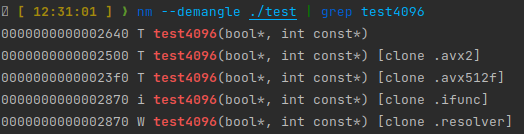## Macro 整合

``````
#ifdef __linux__
#include <sys/auxv.h>
#ifndef HWCAP2_SVE2
#define HWCAP2_SVE2 (1 << 1)
#endif

#ifndef HWCAP_SVE
#define HWCAP_SVE (1 << 22)
#endif

#ifndef AT_HWCAP2
#define AT_HWCAP2 26
#endif

#ifndef AT_HWCAP
#define AT_HWCAP 16
#endif

namespace detail
{
static inline bool sve2_supported()
{
auto hwcaps = getauxval(AT_HWCAP2);
return (hwcaps & HWCAP2_SVE2) != 0;
}

static inline bool sve_supported()
{
auto hwcaps = getauxval(AT_HWCAP);
return (hwcaps & HWCAP_SVE) != 0;
}
} // namespace detail

#endif

#define TMV_STRINGIFY_IMPL(X) #X
#define TMV_STRINGIFY(X) TMV_STRINGIFY_IMPL(X)

#define TIFLASH_MULTIVERSIONED_VECTORIZATION_X86_64(RETURN, NAME, ARG_LIST, ARG_NAMES, BODY)                           \
struct NAME##TiFlashMultiVersion                                                                                   \
{                                                                                                                  \
__attribute__((always_inline)) static inline RETURN inlined_implementation ARG_LIST BODY;                      \
\
__attribute__((target("default"))) static RETURN dispatched_implementation ARG_LIST                            \
{                                                                                                              \
return inlined_implementation ARG_NAMES;                                                                   \
};                                                                                                             \
\
__attribute__((target("avx"))) static RETURN dispatched_implementation ARG_LIST                                \
{                                                                                                              \
return inlined_implementation ARG_NAMES;                                                                   \
};                                                                                                             \
\
__attribute__((target("avx2"))) static RETURN dispatched_implementation ARG_LIST                               \
{                                                                                                              \
return inlined_implementation ARG_NAMES;                                                                   \
};                                                                                                             \
\
__attribute__((target("avx512f,avx512vl,avx512bw,avx512cd"))) static RETURN dispatched_implementation ARG_LIST \
{                                                                                                              \
return inlined_implementation ARG_NAMES;                                                                   \
};                                                                                                             \
\
__attribute__((always_inline)) static inline RETURN invoke ARG_LIST                                            \
{                                                                                                              \
return dispatched_implementation ARG_NAMES;                                                                \
};                                                                                                             \
};

#define TIFLASH_MULTIVERSIONED_VECTORIZATION_AARCH64(RETURN, NAME, ARG_LIST, ARG_NAMES, BODY)     \
struct NAME##TiFlashMultiVersion                                                              \
{                                                                                             \
__attribute__((always_inline)) static inline RETURN inlined_implementation ARG_LIST BODY; \
\
static RETURN generic_implementation ARG_LIST                                             \
{                                                                                         \
return inlined_implementation ARG_NAMES;                                              \
};                                                                                        \
\
__attribute__((target("sve"))) static RETURN sve_implementation ARG_LIST                  \
{                                                                                         \
return inlined_implementation ARG_NAMES;                                              \
};                                                                                        \
\
__attribute__((target("sve2"))) static RETURN sve2_implementation ARG_LIST                \
{                                                                                         \
return inlined_implementation ARG_NAMES;                                              \
};                                                                                        \
\
static RETURN dispatched_implementation ARG_LIST                                          \
__attribute__((ifunc(TMV_STRINGIFY(__tiflash_mvec_##NAME##_resolver))));              \
\
__attribute__((always_inline)) static inline RETURN invoke ARG_LIST                       \
{                                                                                         \
return dispatched_implementation ARG_NAMES;                                           \
};                                                                                        \
};                                                                                            \
extern "C" void * __tiflash_mvec_##NAME##_resolver()                                          \
{                                                                                             \
if (::detail::sve_supported())                                                            \
{                                                                                         \
return reinterpret_cast<void *>(&NAME##TiFlashMultiVersion::sve_implementation);      \
}                                                                                         \
if (::detail::sve2_supported())                                                           \
{                                                                                         \
return reinterpret_cast<void *>(&NAME##TiFlashMultiVersion::sve2_implementation);     \
}                                                                                         \
return reinterpret_cast<void *>(&NAME##TiFlashMultiVersion::generic_implementation);      \
}

#if defined(__linux__) && defined(__aarch64__)
#define TIFLASH_MULTIVERSIONED_VECTORIZATION TIFLASH_MULTIVERSIONED_VECTORIZATION_AARCH64
#elif defined(__linux__) && defined(__x86_64__)
#define TIFLASH_MULTIVERSIONED_VECTORIZATION TIFLASH_MULTIVERSIONED_VECTORIZATION_X86_64
#else
#define TIFLASH_MULTIVERSIONED_VECTORIZATION(RETURN, NAME, ARG_LIST, ARG_NAMES, BODY) \
struct NAME##TiFlashMultiVersion                                                  \
{                                                                                 \
__attribute__((always_inline)) static inline RETURN invoke ARG_LIST BODY;     \
};
#endif

TIFLASH_MULTIVERSIONED_VECTORIZATION(
int,
sum,
(const int * __restrict a, int size),
(a, size),
{
int sum = 0;
for (int i = 0; i < size; ++i) {
sum += a[i];
}
return sum;
}
)
``````

# 面向编译器的优化

LLVM提供了一个很好的自动向量化指南： Auto-Vectorization in LLVM - LLVM 15.0.0git documentation

## 检查向量化条件

• `-Rpass-missed='.*vectorize.*'`检查编译器为什么没有成功向量化
• `-Rpass='.*vectorize.*'`检查编译器进行了那些向量化

``````cat compile_commands.json | grep "/VersionFilterBlockInputStream.cpp"
``````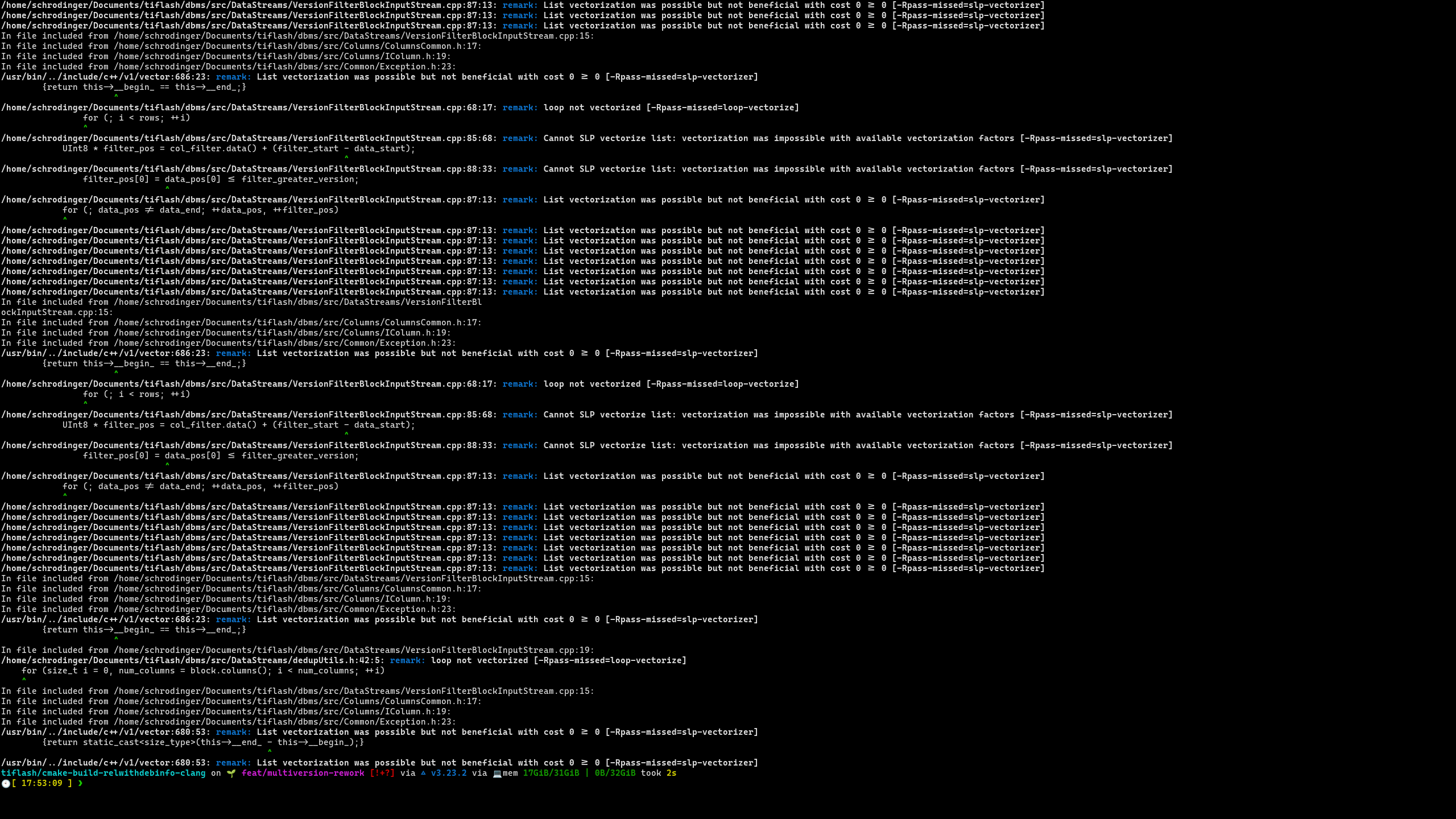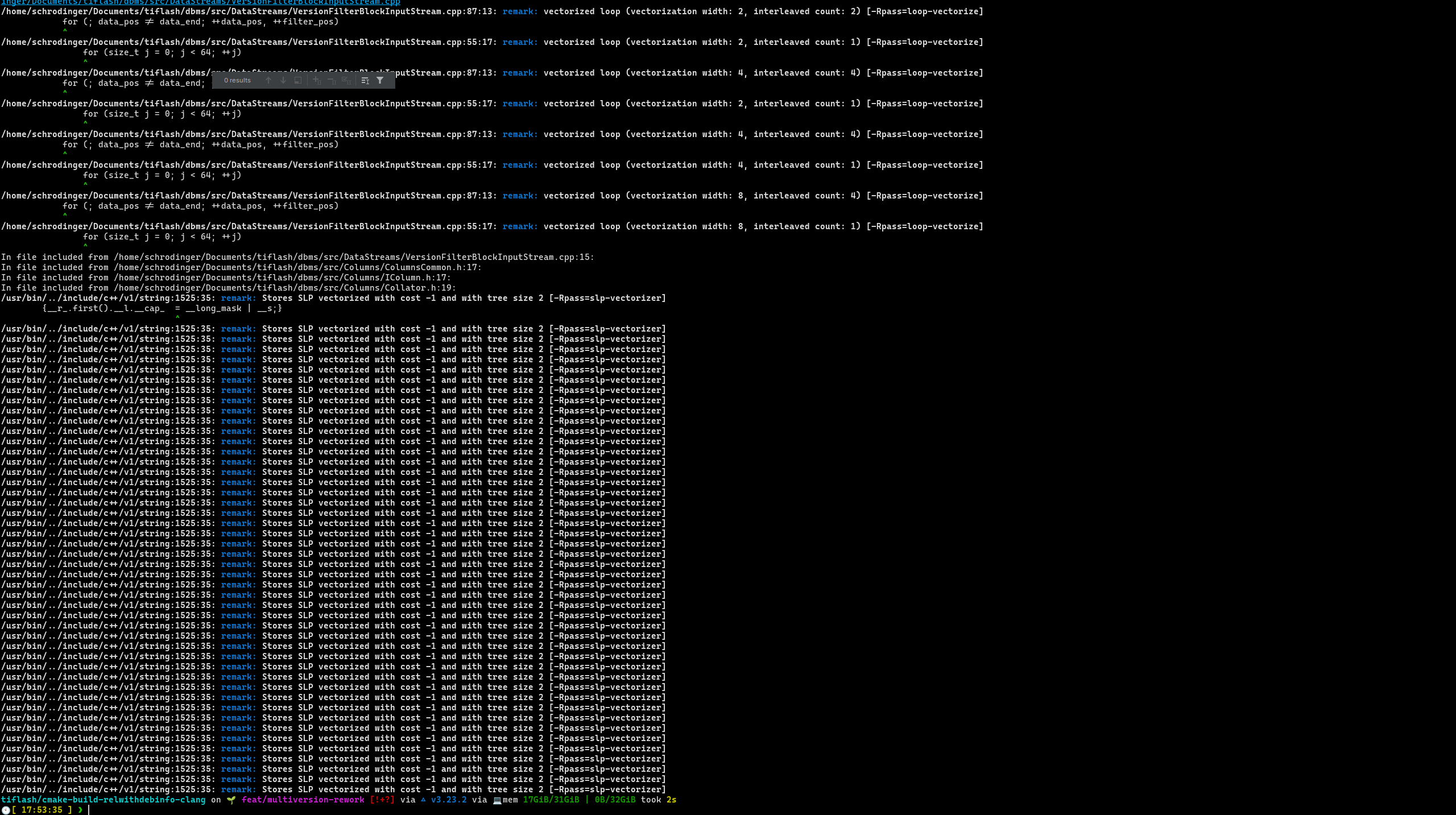## 循环展开 Pragma

``````void test1(int * a, int *b, int *c) {
#pragma clang loop unroll(full)
for(int i = 0; i < 1024; ++i) {
c[i] = a[i] + b[i];
}
}

void test2(int * a, int *b, int *c) {
#pragma clang loop unroll(enable)
for(int i = 0; i < 1024; ++i) {
c[i] = a[i] + b[i];
}
}

void test3(int * a, int *b, int *c) {
#pragma clang loop unroll(disable)
for(int i = 0; i < 1024; ++i) {
c[i] = a[i] + b[i];
}
}

void test4(int * a, int *b, int *c) {
#pragma clang loop unroll_count(2)
for(int i = 0; i < 1024; ++i) {
c[i] = a[i] + b[i];
}
}
``````

## 向量化 Pragma

``````static constexpr int N = 4096;
int A[N];
int B[N];

struct H {
double a;
H operator*(const H& that) {
return {
a * that.a,
a * that.a,
a * that.a,
a * that.a,
};
}
};

H C[N];
H D[N];
H E[N];

void test1() {
#pragma clang loop vectorize(enable)
for (int i=0; i < N; i++) {
C[i] = D[i] * E[i];
}
}

void test2() {
for (int i=0; i < N; i++) {
C[i] = D[i] * E[i];
}
}
``````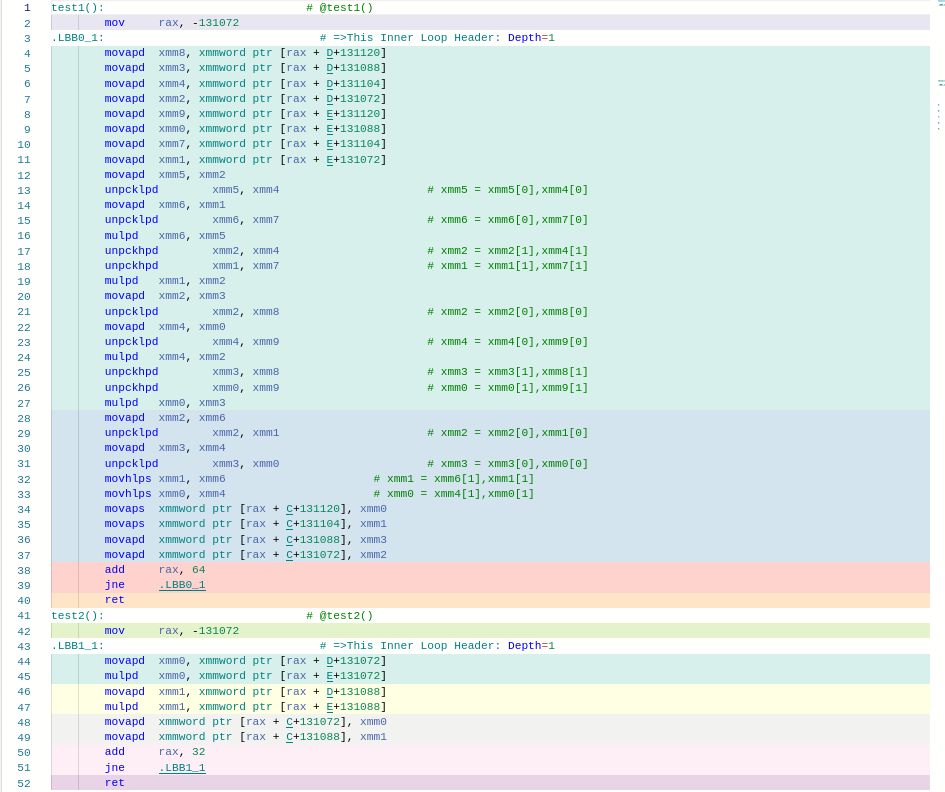## 循环拆分

``````void x() {
#pragma clang loop vectorize(enable)
for (int i=0; i < N; i++) {
A[i + 1] = A[i] + B[i];
C[i] = D[i] * E[i];
}
}

void y() {
for (int i=0; i < N; i++) {
A[i + 1] = A[i] + B[i];
}
#pragma clang loop vectorize(enable)
for (int i=0; i < N; i++) {
C[i] = D[i] * E[i];
}
}
``````

``````#pragma clang loop distribution(enable)
``````

## 控制向量化策略

### 调整单位向量大小

``````void test(char *x, char *y, char * z) {
#pragma clang loop vectorize_width(8)
for (int i=0; i < 4096; i++) {
x[i] = y[i] * z[i];
}
}
``````

### 调整向量化批次大小

``````void test(char *x, char *y, char * z) {
#pragma clang loop vectorize_width(8) interleave_count(4)
for (int i=0; i < 4096; i++) {
x[i] = y[i] * z[i];
}
}
``````

## 提取定长循环单元

``````const uint64_t* filterRow(
const uint64_t* data,
size_t length,
uint64_t current_version) {
for(size_t i = 0; i < length; ++i) {
if (data[i] > current_version) {
return data + i;
}
}
return nullptr;
}
``````

``````const uint64_t* filterRow(
const uint64_t* data,
size_t length,
uint64_t current_version) {
size_t i = 0;

for(; i + 64 < length; i += 64) {
#pragma clang loop vectorize(enable)
for (size_t j = 0; j < 64; ++j) {
mask |= data[i + j] > current_version ? (1ull << j) : 0;
}
return data + i + __builtin_ctzll(mask);
}
}

for(; i < length; ++i) {
if (data[i] > current_version) {
return data + i;
}
}

return nullptr;
}
``````

`__builtin_ctzll` 是用来计算整数末尾0的个数的编译器内建函数，一般可以高效地翻译成一条指令）

0
4
1
1